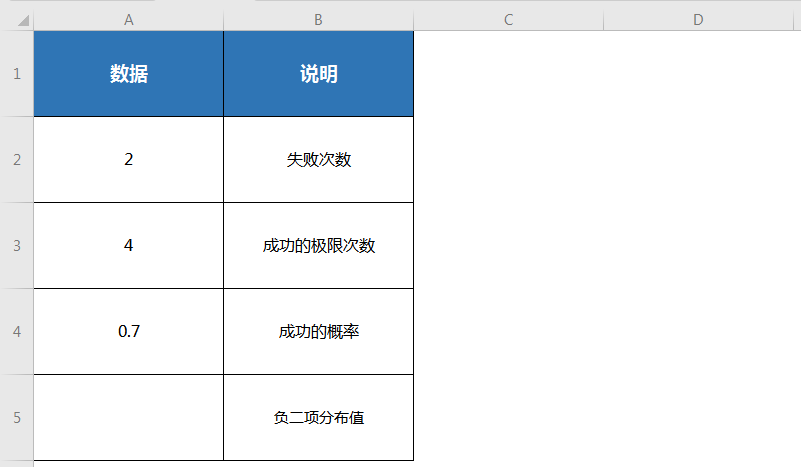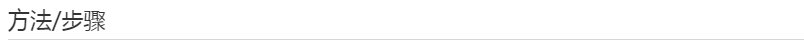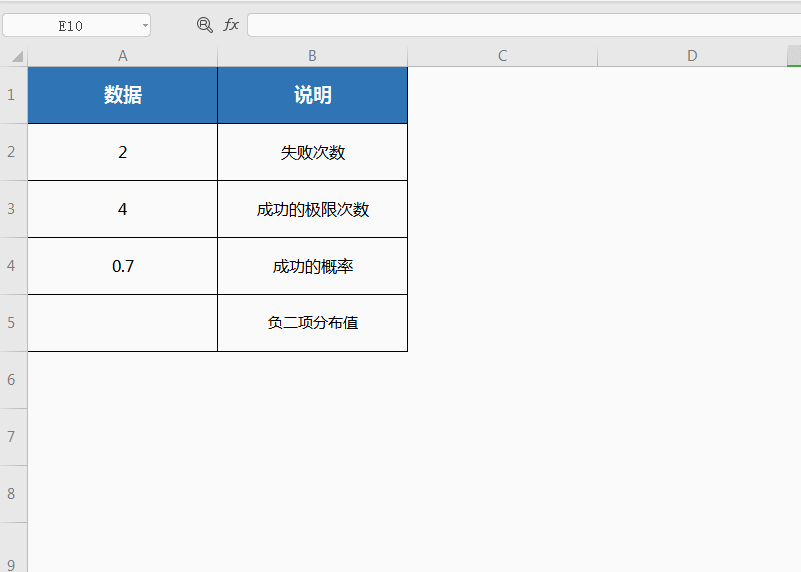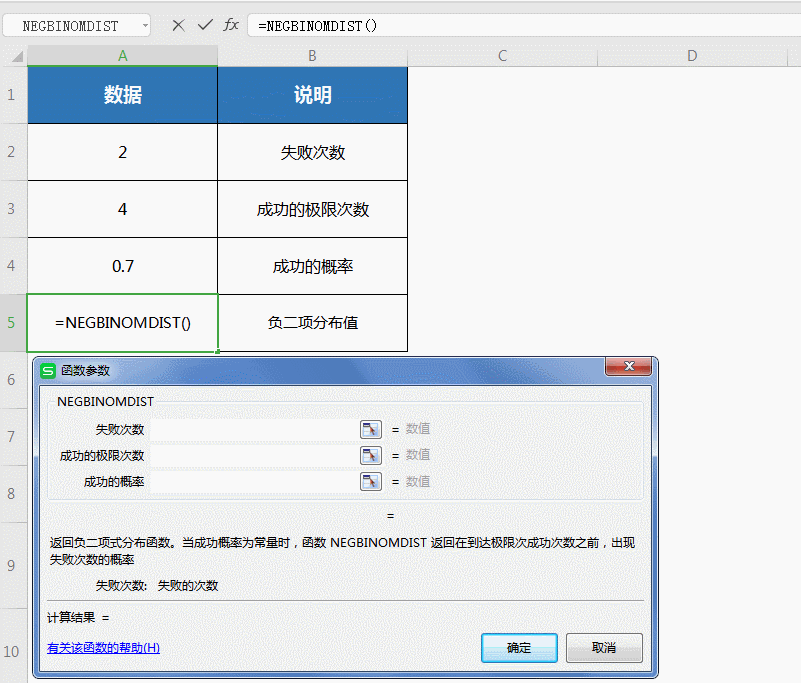## NEGBINOMDIST函数

NEGBINOMDIST(number_f,number_s,probability_s)
Number_f 失败次数。
Number_s 成功的极限次数。
Probability_s 成功的概率。

Number_f 和 number_s 将被截尾取整。x 是 number_f，r 是 number_s，且 p 是 probability_s。￭选中B2单元格，点击插入函数，在查找框中查找并选中NEGBINOMDIST函数，点击确定；￭此时弹出对话框，共三项参数：